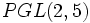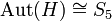# Supergroups of special linear group:SL(2,5)

Jump to: navigation, search
This article gives specific information, namely, supergroups, about a particular group, namely: special linear group:SL(2,5).
View supergroups of particular groups | View other specific information about special linear group:SL(2,5)

This article discusses some of the groups that admit special linear group:SL(2,5) as a subgroup, quotient group, or subquotient.

Note that unlike the discussion of subgroup structure of special linear group:SL(2,5), the discussion is necessarily not comprehensive, because there are infinitely many groups containing any given group.

## Subgroups and quotients: essential minimalist examples

### Subgroups: making all automorphisms inner

The automorphism group of SL(2,5) is isomorphic to symmetric group:S5, which can be more explicitly thought of as PGL(2,5) in this context. Explicitly, the elements of$GL(2,5)$ act as automorphisms of$SL(2,5)$ by conjugation, and the kernel of this action is the center, so this induces a faithful action of$PGL(2,5)$ on$SL(2,5)$ and an embedding of$PGL(2,5)$ inside the automorphism group of$SL(2,5)$. In this case, that embedding is surjective, i.e.,$SL(2,5)$ has no further automorphisms.

There are a number of ways we can find a group$G$ containing a subgroup$H$ isomorphic to$SL(2,5)$ as a normal subgroup so that the induced action by conjugation yields a surjective homomorphism$G/C_G(H) \to \operatorname{Aut}(H)$ (in other words,$G$ contains a normal fully normalized subgroup isomorphic to$SL(2,5)$).

The minimal examples are those where$H$ is a self-centralizing subgroup of$G$ (i.e.,$C_G(H) = Z(H)$), i.e., is a NSCFN-subgroup of$G$. In this case,$G$ has normal subgroup$Z(H)$ of order two, with quotient group$\operatorname{Aut}(H)$ of order 120. In particular,$G$ is a double cover of$\operatorname{Aut}(H) \cong S_5$. In particular,$G$ must have order 240.

The upshot is that the classification of groups that contain SL(2,5) as a NSCFN-subgroup is exactly the same as the classification of double covers of the automorphism group, which is isomorphic to symmetric group:S5. There are two such groups, both of order 240:

In addition, there are many non-minimal examples. For instance, general linear group:GL(2,5) is a group of order 480 containing SL(2,5) as a normal fully normalized subgroup of index four.

### Quotients: Schur covering groups

The group special linear group:SL(2,5) is a Schur-trivial group, i.e., its Schur multiplier is a trivial group. Further, the group is a perfect group. Thus, its Schur covering group is itself.